Electron. J. Diff. Eqns., Vol. 2007(2007), No. 177, pp. 1-9.

### Existence of positive solutions for p(x)-Laplacian problems Ghasem A. Afrouzi, Horieh Ghorbani

Abstract:
We consider the system of differential equations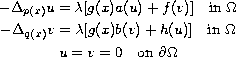where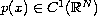is a radial symmetric function such that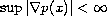,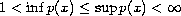, and where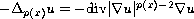which is called the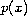-Laplacian. We discuss the existence of positive solution via sub-super-solutions without assuming sign conditions on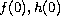.

Submitted July 18, 2007. Published December 17, 2007.
Math Subject Classifications: 35J60, 35B30, 35B40
Key Words: Positive radial solutions; p(x)-Laplacian problems; boundary value problems.

Show me the PDF file (221 KB), TEX file, and other files for this article.Ghasem A. Afrouzi Department of Mathematics Faculty of Basic Sciences Mazandaran University, Babolsar, Iran email: afrouzi@umz.ac.ir Horieh Ghorbani Department of Mathematics Faculty of Basic Sciences Mazandaran University, Babolsar, Iran email: seyed86@yahoo.com